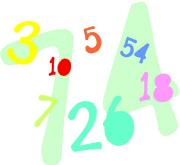## NUMBER SEQUENCES[Preface NOTE: The material presented below was lifted from an article I saw some time ago by Dan Brutlag, "Making Your Own Rules". THE MATHEMATICS TEACHER, November 1990. pp. 608-611. What is being given below is how I prepared a handout to give to my classes, mostly as an activity for use by a substitute.]

# NUMBER SEQUENCES

Students: I found the information below in a math magazine. I think you will find it interesting, as I did.

1. Study/investigate the results of applying the rules below to many different numbers. Keep good records to see if something "special" always happens. Describe what you find.

2. Then make up a set of rules of your own to investigate, similar to or completely different from the samples given. Use the table of ideas that you find at the end of this handout to help you with this. Write up a little report about what you discover.

A. Lori's Rule

2. To get the hundreds digit of the next number in the sequence, take the starting number's hundreds digit and double it. If the double is more than 9, then add the double's digits together to get a one-digit number.
3. Do the same thing to the tens and units digits of the starting number to obtain the tens and units digits of the new number.
4. Repeat Steps 2 and 3 as often as necessary to find the special happening.

Example: 567, 135, 261, 432, ???, ???, ...

B. Barbette's Rule

2. Add all the digits together and multiply by 2 to get the hundreds and tens digits of the next number in the sequence.
3. To get the units digit of the next number, take the starting number's tens and units digits and add them. If the sum is more than 9, add the digits of that sum to get a one-digit number for the units place.
4. Repeat Steps 2 and 3 as often as necessary to find the special happening.
Example: 563, 289, 388, 387, ???, ???, ...

C. Ramona's Rule

2. Obtain the next number in the sequence by moving the hundreds digit of the starting number ito the tens place of the next number, the tens digit of the original number into the units place of the next number, and the units digit into the hundreds place.
3. Then add 2 to the units digit of the new number; however, if the sum is greater than 9, use only the units digit of the sum
4. Repeat Steps 2 and 3 as often as necessary to find the special happening.

Example: 324, 434, 445, 546, ???, ???, ...

D. Lisa's Rule

2. If the number is a multiple of 3, the divide it by 3 to get a new number.
3. If it is not a multiple of 3 then get a new number by squaring the sum of the digits of the number.
4. Repeat Steps 2 and 3 as often as necessary to find the special happening.

Example: 315, 105, 35, 64, ???, ???, ...

Example: 723, 241, 49, 169, 256, ???, ???, ...,

TABLE OF ATTRIBUTE LISTS
 NUMBER OPERATORS NUMBER PROPERTIES Add ___ to ___ Divisible by ___ Move to another position Hundreds, tens, or units place Take positive difference Prime Double Composite Square Even, Odd Halve (if even) Multiple of ___ Multiply by ___ Greater than Replace by ___ Less than Exchange Equal to

### Postscript (7/29/99)

Here is a personal sidelight to this activity. On April 23, 1991, I designed my own sequence rule-set. Here it is.

TROTTER'S RULE